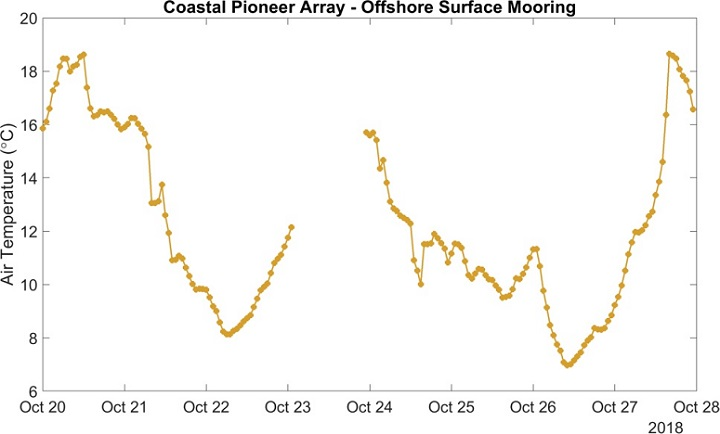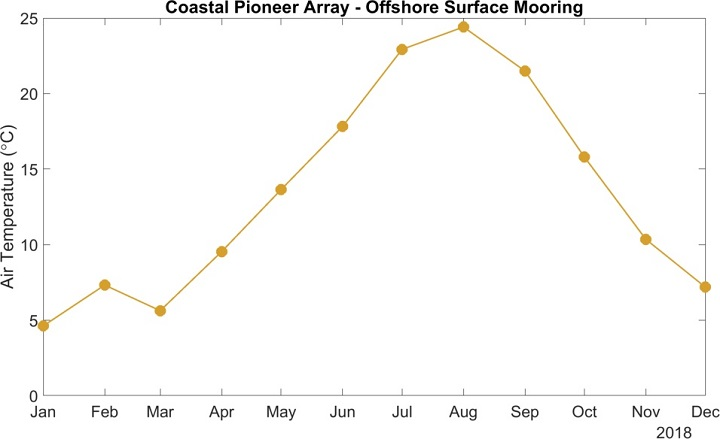## Lab 2.1 – Reading a time series graph

Fundamental concept: Variability in the data, finding trends
Estimated time to complete: 30 minutes
Materials needed (none)

The graph of river discharge in the introduction to Lab 2 is a time series plot and nicely shows the effect of a process, springtime rain. If you were studying the oceanography of the Oregon coast this information would help you interpret the salinity patterns there. Other processes can be revealed in other time series plots. Here we examine temperature data to see what the time series can tell us.Figure 2.1.1

The figure 2.1.1 shows the air temperature as measured at the OOI Coastal Pioneer Offshore mooring. Let’s explore this graph and see if we can make sense of it.

Shown is a year of data. Answer the following questions to become familiar with this time series plot (Figure 2.1.1).

1. What was the start and end date for data collection?
2. What variable is plotted on the y-axis of this graph and what are the units?
3. How does this variable vary throughout the year? Does the variation make sense?
4. A trend in the data is when there is a gradual change, i.e. an increase or decrease, over time, depth or distance. Are there any trends in these data?

The graph (Figure 2.1.2) below shows a small part of the data set you just examined.Figure 2.1.2

1. What is the start and end date of the data collection in Figure 2.1.2?
2. Notice that there is a gap in the data. What does that gap mean?
3. Are there any trends in the data? What do you think causes them?
4. What are the maximum and minimum values in the data?
5. Considering your answers to these questions can you explain the “messiness” of the data in the first graph?

Sometimes it is easier to look at long term data by taking averages, for example it is often useful to average temperature data over a 24 hour period to remove the temperature change that occurs between day and night. For other studies an average of all the data collected during a month may be appropriate. The graph (Figure 2.1.3) below shows the same data set examined in the previous graphs, but the data points are monthly averages of temperature.Figure 2.1.3

1. What are the maximum and minimum temperature values, and what do you think causes them?
2. The graph above (Figure 2.1.3) looks much “neater” than the previous graphs. This is because much of the detail has been lost in the averaging process. Is this a fair trade-off if you want to look at seasonal changes in temperature?
3. Is one or more trend(s) evident in the monthly mean temperature data shown in the figure 2.1.3? Describe the trend(s).

Sometimes we want to compare two data sets to see if they are related. One way to do that is to plot them on the same set of axes. Below is the same graph of air temperature but now the sea surface temperature has been added to it.Figure 2.1.4

1. Based on Figure 2.1.4, are there any trends in the sea surface temperature data? What are they?
2. What is the maximum and minimum in the sea surface temperature and when do they occur (Figure 2.1.4)?
3. How does the seasonal range in sea surface temperature compare to the seasonal range in air temperature? Why do you think that they aren’t the same?
4. Is sea surface temperature correlated with air temperature?

Figure 2.1.5

When scientists want to compare two different types of measurements they can plot them on the same graph, but have to use different y-axes. Examine the graph above, which shows the surface temperature data and surface salinity data. Note that the temperature data are plotted relative to the y-axis on the LEFT, while the salinity data are plotted relative to the y-axis on the RIGHT.

1. What are the maximum and minimum values for sea surface salinity (Figure 2.1.5)?
2. Are there any trends in the salinity data? What are they?
3. Notice that you can click and drag the two scroll bars at the bottom of the graph to zoom in and out to different portions of this graph. Zoom in so that only April and May are visible. Does there appear to be a correlation between sea surface temperature and salinity? If so, what type of relationship did you find between temperature and salinity?
4. Now zoom out to view the rest of the year. Does the same relationship between sea surface salinity and temperature hold for the whole year?

#### Reflection

Real data often looks “messy”. It becomes neater if one averages the data, but this removes the information about any variability within the averaged data. If you were to take all of the salinity data collected at a river mouth and create yearly averages (i.e. average all the data for each calendar year) what kind of detail do you think you will lose? In other words, what natural processes will be lost in the averaging? What might you gain from doing such an average?